Methods and formulas for Predict

Select the method or formula of your choice.

Confidence interval

The range in which the estimated mean response for a given set of predictor values is expected to fall.

Notation

TermDescription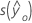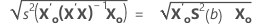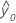fitted response value for a given set of predictor values
α type I error rate
n number of observations
p number of model parameters
S 2(b)variance-covariance matrix of the coefficients
s 2 mean square error
X design matrix
X0 vector of given predictor values
x'0transpose of the new vector of predictor values

Fit

Notation

TermDescription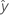fitted value
xkkth term. Each term can be a single predictor, a polynomial term, or an interaction term.
bkestimate of kth regression coefficient

Prediction interval

The prediction interval is the range in which the fitted response for a new observation is expected to fall.

Notation

TermDescription
s(Pred)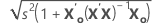fitted response value for a given set of predictor values
α level of significance
n number of observations
p number of model parameters
s 2 mean square error
X predictor matrix
X0 matrix of given predictor values
x'0transpose of the new vector of predictor values

Regression equation

For a model with multiple predictors, the equation is:

y = β0 + β1x1 + … + βkxk + ε

The fitted equation is:

In simple linear regression, which includes only one predictor, the model is:

y=ß0+ ß1x1+ε

Using regression estimates b0 for ß0, and b1 for ß1, the fitted equation is:

Notation

TermDescription
yresponse
xkkth term. Each term can be a single predictor, a polynomial term, or an interaction term.
ßkkth population regression coefficient
εerror term that follows a normal distribution with a mean of 0
bkestimate of kth population regression coefficientfitted response

Standard error of fitted value (SE Fit)

The standard error of the fitted value in a regression model with one predictor is:

The standard error of the fitted value in a regression model with more than one predictor is:

Notation

TermDescription
s2mean square error
nnumber of observations
x0new value of the predictor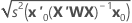mean of the predictor
xiith predictor value
x0 vector of values that produce the fitted values, one for each column in the design matrix, beginning with a 1 for the constant term
x'0transpose of the new vector of predictor values
Xdesign matrix
By using this site you agree to the use of cookies for analytics and personalized content.  Read our policy